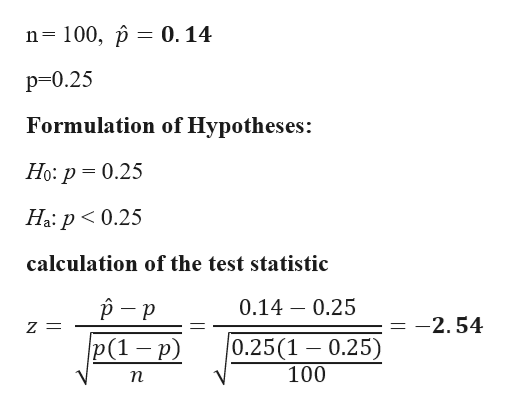# Use the normal distribution and the given sample results to complete the test of the given hypotheses. Assume the results come from a random sample and use a 5% significance level.Test H0 : p=0.25 vs Ha : p<0.25 using the sample results p^=0.14 with n=100Round your answer for the test statistic to two decimal places, and your answer for the p-value to three decimal places.Test Statistic=p-value=

Question
1 views

Use the normal distribution and the given sample results to complete the test of the given hypotheses. Assume the results come from a random sample and use a 5% significance level.

Test H0 : p=0.25 vs Ha : p<0.25 using the sample results p^=0.14 with n=100

Test Statistic=

p-value=

check_circle

Step 1

Given Dat...help_outlineImage Transcriptionclosen= 100, p = 0. 14 p=0.25 Formulation of Hypotheses: Но: р 3 0.25 Ha: p< 0.25 calculation of the test statistic 0.14 – 0.25 = -2.54 p(1 – p) 0.25(1 – 0.25) 100 п fullscreen

### Want to see the full answer?

See Solution

#### Want to see this answer and more?

Solutions are written by subject experts who are available 24/7. Questions are typically answered within 1 hour.*

See Solution
*Response times may vary by subject and question.
Tagged in

### Hypothesis Testing